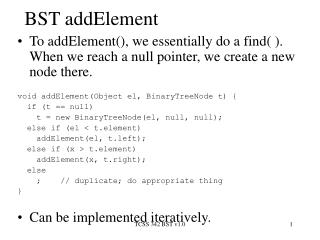DownloadDownload PresentationTélécharger la présentation- - - - - - - - - - - - - - - - - - - - - - - - - - - E N D - - - - - - - - - - - - - - - - - - - - - - - - - - -
##### Presentation Transcript

1. BST addElement • To addElement(), we essentially do a find( ). When we reach a null pointer, we create a new node there. void addElement(Object el, BinaryTreeNode t) { if (t == null) t = new BinaryTreeNode(el, null, null); else if (el < t.element) addElement(el, t.left); else if (x > t.element) addElement(x, t.right); else ; // duplicate; do appropriate thing } • Can be implemented iteratively. TCSS 342 BST v1.0

2. 5 4 8 1 7 11 3 findMin, findMax • To find the maximum element in the BST, we follow right children until we reach NULL. • To find the minimum element in the BST, we follow left children until we reach NULL. TCSS 342 BST v1.0

3. BST remove • Removing an item disrupts the tree structure. • Basic idea: find the node that is to be removed. Then “fix” the tree so that it is still a binary search tree. • Three cases: • node has no children • node has one child • node has two children TCSS 342 BST v1.0

4. 5 4 8 1 7 11 3 5 4 8 1 7 11 3 No children, one child TCSS 342 BST v1.0

5. 5 4 8 1 7 11 3 7 4 8 1 7 11 3 Two children • Replace the node with its successor. Then remove the successor from the tree. TCSS 342 BST v1.0

6. Height of BSTs • n-node BST: Worst case depth: n-1. • Claim: The maximum number of nodes in a binary tree of height h is 2h+1 – 1. Proof: The proof is by induction on h. For h = 0, the tree has one node, which is equal to 20+1 – 1. Suppose the claim is true for any tree of height h. Any tree of height h+1 has at most two subtrees of height h. By the induction hypothesis, this tree has at most 2(2h+1 – 1)+1 = 2h+2 – 1. TCSS 342 BST v1.0

7. Height of BSTs, cont’d • If we have a BST of n nodes and height h, then by the Claim, n 2h+1 – 1. So, h log (n+1) – 1. • Average depth of nodes in a tree. Assumptions: insert items randomly (with equal likelihood); each item is equally likely to be looked up. • Internal path length: the sum of the depths of all nodes. TCSS 342 BST v1.0

8. r TR TL Average Depth • Let D(n) be the internal path length of some tree with n nodes. • The left subtree has i nodes, and the right subtree has n–i–1 nodes. • D(1) = 0 • D(n) = D(i) + D(n – i – 1) + n – 1 TCSS 342 BST v1.0

9. D(n)  1.442 n log n • How long does it take to insert the items 1, 2, 3, …, n (in that order) into a BST? • What if they were inserted so that the resulting BST were perfectly balanced? TCSS 342 BST v1.0